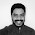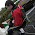Skip to main content

### Random Bouncing Ball Animation

Simple animation of random balls that bounces within the screen

Source:

``````#include<stdio.h>
#include<graphics.h>
#include<conio.h>
#include<dos.h>
#include<math.h>

void drawBall(struct ball *b, int color);

struct ball{
int x, y;
int dx, dy;
int radius;
};

void main()
{
int gd=0, gm=VGAHI;
int i;
struct ball b;
initgraph(&gd, &gm, "");

for(i=1;i<=15;i++){
b[i].radius = rand()%20;
b[i].x=rand()%getmaxx();
b[i].y=rand()%getmaxy();
b[i].dx=2;
b[i].dy=4;
}

while(!kbhit())
{
delay(5);
cleardevice();
for(i=1;i<=15;i++)
drawBall(&b[i],i);
}
closegraph();

}

void drawBall(struct ball *b, int color){
setfillstyle(1,color);
setcolor(color);
fillellipse(b->x, b->y, b->radius, b->radius);
if(b->x+b->radius > getmaxx() || b->x-b->radius<0)
b->dx = -b->dx;
if(b->y+b->radius > getmaxy() || b->y-b->radius<0)
b->dy = -b->dy;

b->x+=b->dx;
b->y+=b->dy;
}
```
```

Output:

### Comments

1.can u tell the if statement explanation...please i need to understand it

2.Hi sumbul risvi,

if statement is to check whether the ball is touching edges of the wall

getmaxx() -> screen maxX
getmaxy() -> screen maxY

(b->x + b->radius)->right edge of the ball
(b->y + b->radius)->bottom edge of the ball
(b->x - b->radius)->left edge of the ball
(b->y - b->radius)->top edge of the ball

3.okay thank you

4.nice tutorials and samples

great work on c graphics samples

thanks ajai for sharing

5.What Compiler do you use for this program and is the header file "graphics.h" included in the compiler or did you have to make it yourself?
luke

6.I'm using Turbo C3. 'graphics.h' is already included. you have to enable Graphics libraries via 'options>linker>libraries'

### 2D Reflection

#include <stdio.h> #include <stdlib.h> #include<graphics.h> #include<conio.h> #include<math.h> void draw2d(int,int [],int [],int,int); void main() { int gd=DETECT,gm; int x,y,x1,y1,tx=0,ty=0,i,fs; initgraph(&gd,&gm,""); printf("No of sides : "); scanf("%d",&fs); printf("Co-ordinates : "); for(i=0;i<fs;i++) { printf("(x%d,y%d)",i,i); scanf("%d%d",&x[i],&y[i]); } draw2d(fs,x,y,tx,ty); printf("translation (x,y) : "); scanf("%d%d",&tx,&ty); while(1) { clrscr(); cleardevice(); printf("1. X Reflection\n\n2. Y Reflection\n\n3. Exit"); draw2d(fs,x,y,0,0); switch(getche()) { case '1': for(i=0;i<fs;i++) { x1[i]=(tx+((x[i]-tx)*cos(M_PI))-((y[i]-ty)*sin(M_PI))); y1[i]=y[i]; } break; case '2': for(i=0;i<fs;i++) { x1[i]=x[i]; y1[i]=(tx+((y[i]-ty)*cos(M_PI))+((x[i]-tx)*sin(M_PI))); } break; case '3': close…

### Simple Animation Using C

Here i have used fillpoly function to draw the object body and used fillellipse function to draw tier.
animation is done by looping through the objects x & y position until user hits a key. Keypress event is achived  by using kbhit function. Smoothness of animation is controlled by delay function.

Change the delay values to change the animation speed

Source:

#include<stdio.h> #include<conio.h> #include<graphics.h> #include<dos.h> void main() { int gd=DETECT,gm,i=-300,j; int poly={100,100,250,100,250,50,300,50,325,90,325,140,100,140,100,100}; int tpoly={100,100,250,100,250,50,300,50,325,90,325,140,100,140,100,100}; initgraph(&gd,&gm,""); getch(); while(!kbhit()) { for(j=0;j<16;j+=2) { poly[j]=tpoly[j]+i; } fillpoly(8,poly); setfillstyle(5,7); bar(275+i,60,295+i,85); setfillstyle(5,8); fillellipse(140+i,140,20,20); fillellipse(280+i,140,20,20); setfillstyle(1,0); fillellipse(140+i,140,10,10); fillellipse(280+i,140,10,10); setcolor(…

### Personal Diary - A Mini Project Written in Turbo C With Graphical Interface

A Simple Personal Diary written in Turbo C with Graphical User Interface

Features,
Birthday ReminderNotesAddress Book The main part of the project is UI

'GUI.h' header file includes texbox, message-box and more custom graphical functions
' Dairy.h' header file includes functionality of Personal Dairy
' Dairyload.c' includes loadingscreen, login screen, installation check etc
'Dairy.c' - execution stats here